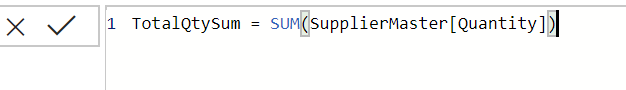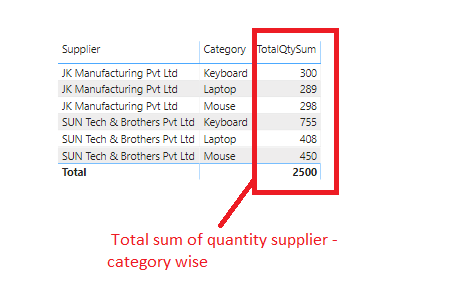Home » IF Function DAX

# IF Function DAXIF function is a Power BI logical function in DAX that checks whether a condition is satisfied, and returns one value in case of TRUE else another value in case of FALSE.

It returns a single value of any type.

If the value referenced in the expression is a column, IF returns the value that corresponds to the current row.

Syntax

`IF(<logical_test>,<value_if_true>[, <value_if_false>])`

<logical_test> is any value or an expression that can be evaluated to TRUE or FALSE.

<value_if_true> is a value that is returned if the <logical_test> is TRUE.

<value_if_false> is a value that is returned if <logical_test> is FALSE. It is an optional.

Lets look at an example, here we have a sample table which consists a supplier data as shown below.Suppose, you want to display the status that is Level1 and Level 2 based on total quantity by Category.

If the total quantity is greater than 500 then display  “Level 1”  else “Level 2”. So, first you need to get the total quantity by supplier and category.

For this, lets create a measure TotalQtySum which calculates the sum of quantity.As you can see, TotalQtySum calculates the sum of quantity.Now, to display the status we will check the total quantity value, if  the total quantity is greater than 500 then display “Level 1”  else “Level 2”.

Lets create a following measure which uses IF function to check the condition.

`Status = IF( [TotalQtySum] >500 ,"Level 1" ,"Level 2" )`Lets drag the measure into table visual to see the output.

As you can see, it returns the status value Level 1 where TotalQtySum is greater than 500 else Level 2.This site uses Akismet to reduce spam. Learn how your comment data is processed.